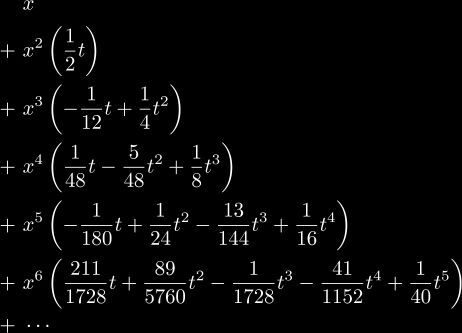Tetration

Featured Pages:  Applications  |  Chaos  |  Exp. Factorial  |  Functional Eq.  |  History  |  Iteration

Reference:  Definitions  |  Identities  |  Methods  |  Code  |  Tables  |  Graphs  |  Glossary  |  Links

Discussion:  Welcome => Basics => Hyperops => Tetration => Super-log => Super-root => Papers

# Iteration Classification

Iteration is fundamental to dynamics, chaos, analysis, recursive functions, and number theory. In most cases the kind of iteration required in these subjects is integer iteration, i.e. where the iteration parameter is an integer. However, in the study of dynamical systems continuous iteration is paramount to the solution of some systems. Different kinds of iteration can be classified as follows:

• Discrete Iteration
• Integer Iteration
• The usual definition of iteration, where the functional equation:
f n(x) = f(f n-1(x))
is used to generate the sequence:
{f(x), f 2(x), f 3(x), ...}
known as the natural iterates of f(x), which forms a monoid under composition.
For invertible functions f(x), the inverses are also considered iterates, and form the sequence:
{..., f -2(x), f -1(x), x, f(x), f 2(x), ...}
known as the integer iterates of f(x), which forms a group under composition.
• Fractional Iteration or Rational Iteration
• Solving the functional equation: f(x) = gn(x). Once this functional equation is solved,
then the rational iterates f (m/n)(x) are the integer iterates of g(x).
• Non-analytic Fractional Iteration
• By chosing a non-analytic fractional iterate, there is no uniqueness of the solutions obtained. (Iga's method)
• Analytic Fractional Iteration
• By solving for an analytic fractional iterate, there is a unique solution obtained in this way. (Dahl's method)
• Continuous Iteration
• A generalization of the usual notion of iteration, where the functional equation (FE):
f n(x) = f(f n-1(x))
must be satisfied for all n in the domain (real or complex). If this is not the case, then to discuss these kinds of "iteration" (even though they are not technically "iteration" since they do not obey the FE of iteration), we will talk about them as though they were expressions for f n(x) where 0 ≤ Re(n) ≤ 1 and defined elsewhere by the FE of iteration. So even though a method is analytic, if it doesn't satisfy this fundamental FE, then by this re-definition, it is non-analytic.
• Non-analytic Continuous Iteration
• By choosing a non-analytic continuous iterate, there is no uniqueness of the solutions obtained. (Galidakis' and Woon's methods)
• Analytic Continuous Iteration or just Analytic Iteration
• By solving for an analytic continuous iterate, there is a unique solution obtained in this way.
• Real-analytic Iteration
• Complex-analytic Iteration or Holomorphic Iteration

# Known Analytic Iterates

bla

 f n(x) = x + cn if f(x) = x + c (addition) f n(x) = xcn if f(x) = xc (multiplication) f n(x) = x^(c^n) if f(x) = xc (powers) f n(x) = (Ax + B)/(Cx + D) if f(x) = (ax + b)/(cx + d) (linear fractional transformations) where: f n(x) = de(n, x) if f(x) = ex - 1 (decremented exponential) where de(t, x) =Visited: times, last updated: 2006-02-15, by: Andrew Robbins, contact: and_j_rob(at)yahoo(dot)com